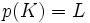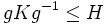# Classifying finite subgroups of a group

This is a survey article related to:subgroups
View other survey articles about subgroups

This article explores the interesting question: given an infinite group, how do we classify all finite subgroups of the group? we'll mainly be looking at linear groups and groups that arise in geometric situations; for instance, fundamental groups or isometry groups of metric spaces and Riemannian manifolds.

## General tactics: pass to easier subgroups, quotients and covers

### Pass to and from subgroups

Suppose$H$ is a subgroup in a group$G$. Then we have a natural map from the finite subgroups of$G$, to the finite subgroups of$H$, given by intersecting with$H$:$K \mapsto K \cap H$

This map is surjective, because any finite subgroup of$H$ is also a finite subgroup of$G$. Thus:

• Going from$G$ to$H$: To classify the finite subgroups of$H$, it suffices to first classify the finite subgroups of$G$, and then check which of them lie inside$H$.
• Going from$H$ to$G$: if we know all the finite subgroups of$H$, then we can, for each finite subgroup$L \le H$, try to determine all the possibilities for a finite subgroup$K$ of$G$ such that$K \cap H = L$. This may not always be easy, but in some cases, it is not hard. For instance, if$H$ is a subgroup of index two in$G$, then any pre-image of$L$ that is not itself$L$, is generated by$L$ and exactly one element in$G \setminus H$. A bit of manipulation can put strong restrictions on what such an element must look like. More generally, the problem is tractable if$H$ is a subgroup of finite index.

### Pass to and from quotients by finite normal subgroups

Suppose$N$ is a normal subgroup of$G$, and$H = G/N$ is the quotient group with the quotient map$p:G \to H$. We have a natural map from finite subgroups of$G$ to subgroups of$H$:$K \mapsto p(K)$

This map is surjective, because for any finite subgroup$L$ of$H$, we can take the full inverse image$p^{-1}(L)$. Thus:

• Going from$G$ to$H$: To classify the finite subgroups of$H$, it suffices to classify the finite subgroups of$G$, and then take the image under$p$
• Going from$H$ to$G$: If we know all the finite subgroups of$H$, then for each finite subgroup$L \le H$, try to determine all the possible finite$K$ such that$p(K) = L$. This is not always easy, but it can be achievable in some cases, particularly the case where$N$ is a finite normal subgroup.

## Finding finite-dominating subgroups

### What's a finite-dominating subgroup?

A subgroup$H$ of a group$G$ is a finite-dominating subgroup if given any finite subgroup$K$ of$G$,$K$ is a subconjugate subgroup of$H$: in other words, there exists$g \in G$ such that$gKg^{-1} \le H$.

If$H$ is a finite-dominating subgroup in$G$, then we can reduce the problem of classifying finite subgroups of$G$, to the problem of classifying finite subgroups of$H$. Here's how:

• Classify all finite subgroups of$H$
• Then, the finite subgroups of$G$ are simply all the subgroups that can be expressed as conjugate subgroups to the finite subgroups of$H$.